# Thermodynamics An Engineering Approach Seventh Edition in SI

• Slides: 24
Download presentation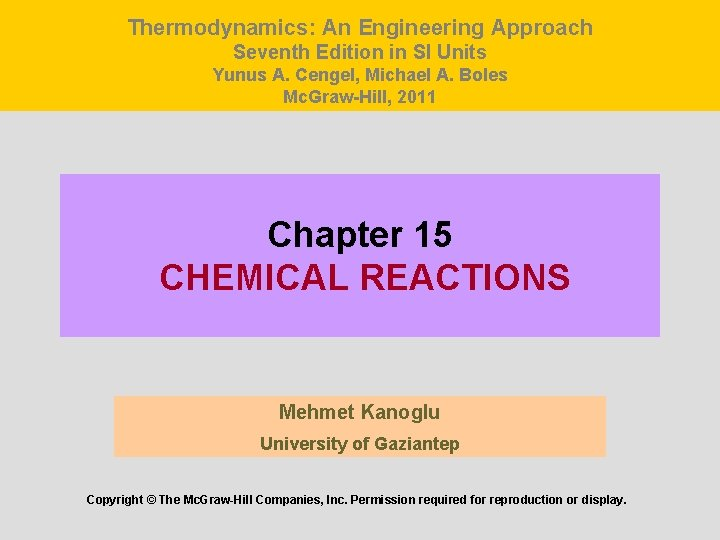Thermodynamics: An Engineering Approach Seventh Edition in SI Units Yunus A. Cengel, Michael A. Boles Mc. Graw-Hill, 2011 Chapter 15 CHEMICAL REACTIONS Mehmet Kanoglu University of Gaziantep Copyright © The Mc. Graw-Hill Companies, Inc. Permission required for reproduction or display.Objectives • Give an overview of fuels and combustion. • Apply the conservation of mass to reacting systems to determine balanced reaction equations. • Define the parameters used in combustion analysis, such as air–fuel ratio, percent theoretical air, and dew-point temperature. • Apply energy balances to reacting systems for both steadyflow control volumes and fixed mass systems. • Calculate the enthalpy of reaction, enthalpy of combustion, and the heating values of fuels. • Determine the adiabatic flame temperature for reacting mixtures. • Evaluate the entropy change of reacting systems. • Analyze reacting systems from the second-law perspective. 2FUELS AND COMBUSTION Fuel: Any material that can be burned to release thermal energy. Most familiar fuels consist primarily of hydrogen and carbon. They are called hydrocarbon fuels and are denoted by the general formula Cn. Hm. Hydrocarbon fuels exist in all phases, some examples being coal, gasoline (usually treated as octane C 8 H 18), and natural gas. Most liquid hydrocarbon fuels are obtained from crude oil by distillation. 3The oxidizer most often used in combustion processes is air. Why? On a mole or a volume basis, dry air is composed of 20. 9% O 2, 78. 1% N 2, 0. 9% Ar, and small amounts of CO 2, He, Ne, H 2. In the analysis of combustion processes, dry air is approximated as 21% O 2 and 79% N 2 by mole numbers. Combustion is a chemical reaction during which a fuel is oxidized and a large quantity of energy is released. Each kmol of O 2 in air is accompanied by 3. 76 kmol of N 2. 4The fuel must be brought above its ignition temperature to start the combustion. The minimum ignition temperatures in atmospheric air are approximately 260°C for gasoline, 400°C for carbon, 580°C for hydrogen, 610°C for carbon monoxide, and 630°C for methane. Proportions of the fuel and air must be in the proper range for combustion to begin. For example, natural gas does not burn in air in concentrations less than 5% or greater than about 15%. The mass (and number of atoms) of each element is conserved during a chemical reaction. The total number of moles is not conserved during a chemical reaction. In a steady-flow combustion process, the components that enter the reaction chamber are called reactants and the components that exit are called products. 5Air-fuel ratio (AF) is usually expressed on a mass basis and is defined as the ratio of the mass of air to the mass of fuel for a combustion process m mass N number of moles M molar mass Fuel–air ratio (FA): The reciprocal of air–fuel ratio. The air–fuel ratio (AF) represents the amount of air used per unit mass of fuel during a combustion process. 6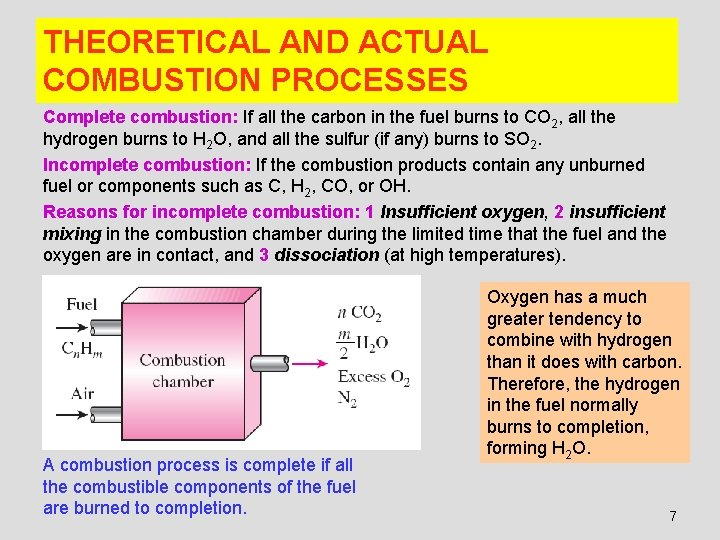THEORETICAL AND ACTUAL COMBUSTION PROCESSES Complete combustion: If all the carbon in the fuel burns to CO 2, all the hydrogen burns to H 2 O, and all the sulfur (if any) burns to SO 2. Incomplete combustion: If the combustion products contain any unburned fuel or components such as C, H 2, CO, or OH. Reasons for incomplete combustion: 1 Insufficient oxygen, 2 insufficient mixing in the combustion chamber during the limited time that the fuel and the oxygen are in contact, and 3 dissociation (at high temperatures). A combustion process is complete if all the combustible components of the fuel are burned to completion. Oxygen has a much greater tendency to combine with hydrogen than it does with carbon. Therefore, the hydrogen in the fuel normally burns to completion, forming H 2 O. 7Stoichiometric or theoretical air: The minimum amount of air needed for the complete combustion of a fuel. Also referred to as the chemically correct amount of air, or 100% theoretical air. Stoichiometric or theoretical combustion: The ideal combustion process during which a fuel is burned completely with theoretical air. Excess air: The amount of air in excess of the stoichiometric amount. Usually expressed in terms of the stoichiometric air as percent excess air or percent theoretical air. Deficiency of air: Amounts of air less than the stoichiometric amount. Often expressed as percent deficiency of air. Equivalence ratio: The ratio of the actual fuel–air ratio to the stoichiometric fuel–air ratio. 50% excess air = 150% theoretical air 200% excess air = 300% theoretical air. 90% theoretical air = 10% deficiency of air The complete combustion process with no free oxygen in the products is called theoretical combustion. 8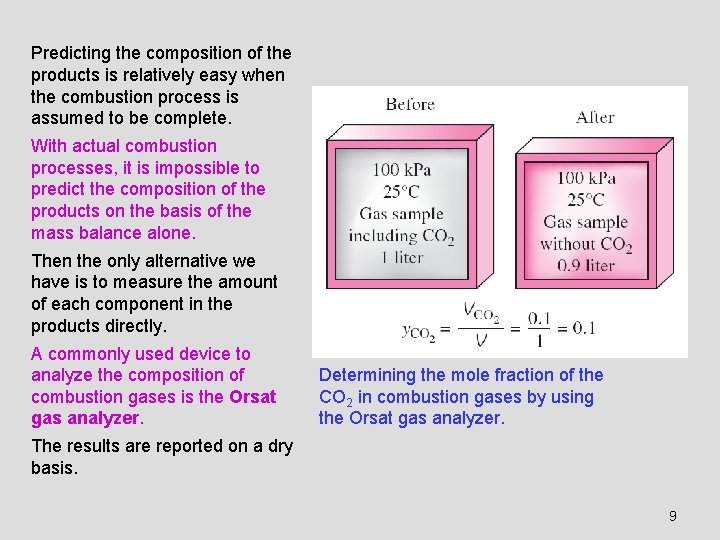Predicting the composition of the products is relatively easy when the combustion process is assumed to be complete. With actual combustion processes, it is impossible to predict the composition of the products on the basis of the mass balance alone. Then the only alternative we have is to measure the amount of each component in the products directly. A commonly used device to analyze the composition of combustion gases is the Orsat gas analyzer. Determining the mole fraction of the CO 2 in combustion gases by using the Orsat gas analyzer. The results are reported on a dry basis. 9ENTHALPY OF FORMATION AND ENTHALPY OF COMBUSTION Disregarding any changes in kinetic and potential energies, the energy change of a system during a chemical reaction is due to a change in state and a change in chemical composition: The microscopic form of energy of a substance consists of sensible, latent, chemical, and nuclear energies. When the existing chemical bonds are destroyed and new ones are formed during a combustion process, usually a large amount of sensible energy is absorbed or released. 10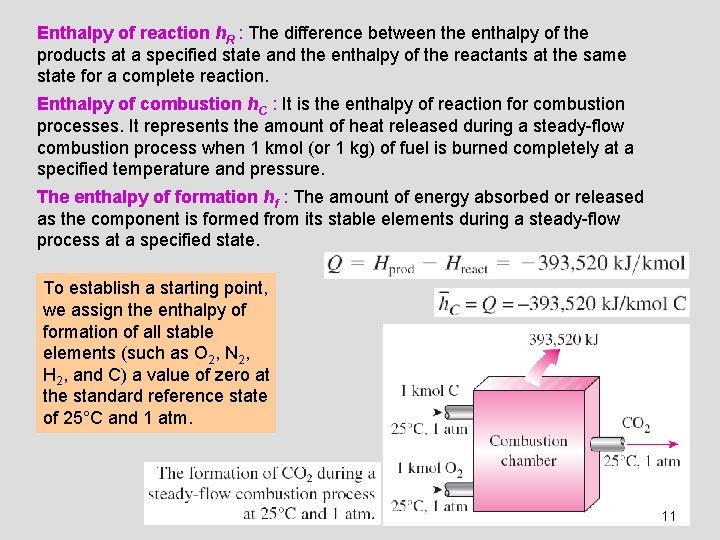Enthalpy of reaction h. R : The difference between the enthalpy of the products at a specified state and the enthalpy of the reactants at the same state for a complete reaction. Enthalpy of combustion h. C : It is the enthalpy of reaction for combustion processes. It represents the amount of heat released during a steady-flow combustion process when 1 kmol (or 1 kg) of fuel is burned completely at a specified temperature and pressure. The enthalpy of formation hf : The amount of energy absorbed or released as the component is formed from its stable elements during a steady-flow process at a specified state. To establish a starting point, we assign the enthalpy of formation of all stable elements (such as O 2, N 2, H 2, and C) a value of zero at the standard reference state of 25°C and 1 atm. 1112Enthalpy of formation: The enthalpy of a substance at a specified state due to its chemical composition. 13Heating value: The amount of heat released when a fuel is burned completely in a steady-flow process and the products are returned to the state of the reactants. The heating value of a fuel is equal to the absolute value of the enthalpy of combustion of the fuel. Higher heating value (HHV): When the H 2 O in the products is in the liquid form. Lower heating value (LHV): When the H 2 O in the products is in the vapor form. The higher heating value of a fuel is equal to the sum of the lower heating value of the fuel and the latent heat of vaporization of the H 2 O in the products. For the fuels with variable composition (i. e. , coal, natural gas, fuel oil), the heating value may be determined by burning them directly in a bomb calorimeter. 14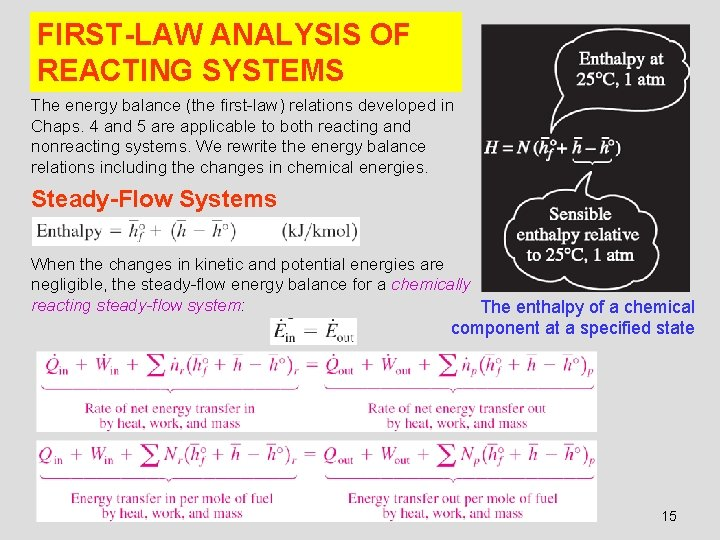FIRST-LAW ANALYSIS OF REACTING SYSTEMS The energy balance (the first-law) relations developed in Chaps. 4 and 5 are applicable to both reacting and nonreacting systems. We rewrite the energy balance relations including the changes in chemical energies. Steady-Flow Systems When the changes in kinetic and potential energies are negligible, the steady-flow energy balance for a chemically reacting steady-flow system: The enthalpy of a chemical component at a specified state 15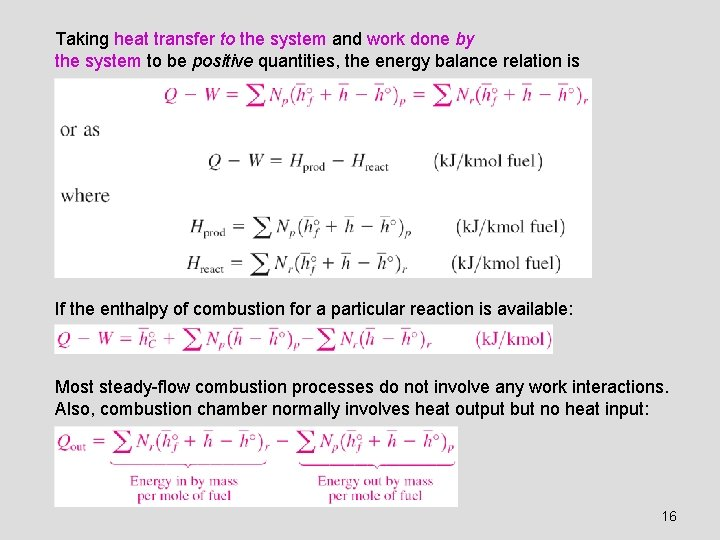Taking heat transfer to the system and work done by the system to be positive quantities, the energy balance relation is If the enthalpy of combustion for a particular reaction is available: Most steady-flow combustion processes do not involve any work interactions. Also, combustion chamber normally involves heat output but no heat input: 16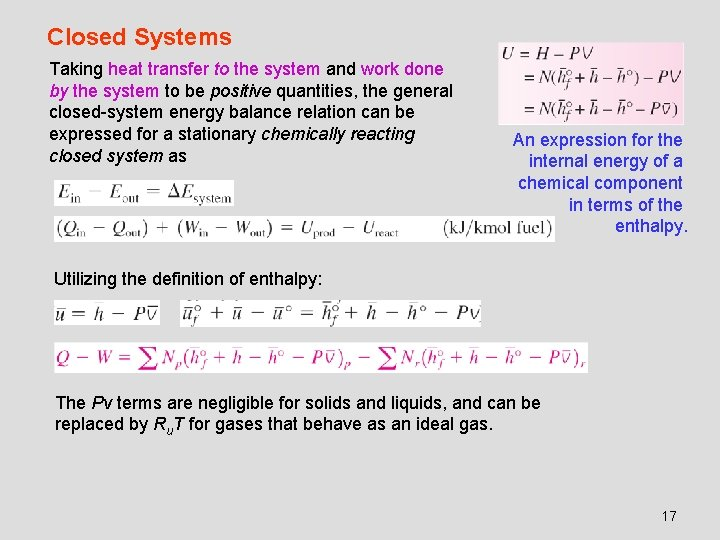Closed Systems Taking heat transfer to the system and work done by the system to be positive quantities, the general closed-system energy balance relation can be expressed for a stationary chemically reacting closed system as An expression for the internal energy of a chemical component in terms of the enthalpy. Utilizing the definition of enthalpy: The Pv terms are negligible for solids and liquids, and can be replaced by Ru. T for gases that behave as an ideal gas. 17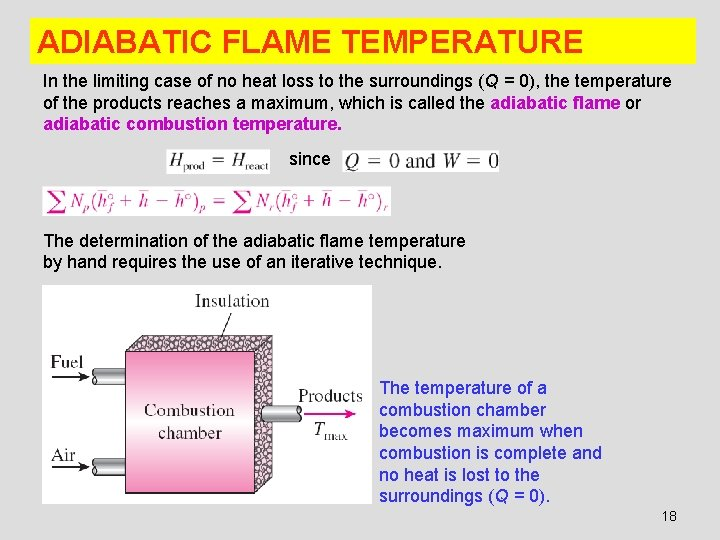ADIABATIC FLAME TEMPERATURE In the limiting case of no heat loss to the surroundings (Q = 0), the temperature of the products reaches a maximum, which is called the adiabatic flame or adiabatic combustion temperature. since The determination of the adiabatic flame temperature by hand requires the use of an iterative technique. The temperature of a combustion chamber becomes maximum when combustion is complete and no heat is lost to the surroundings (Q = 0). 18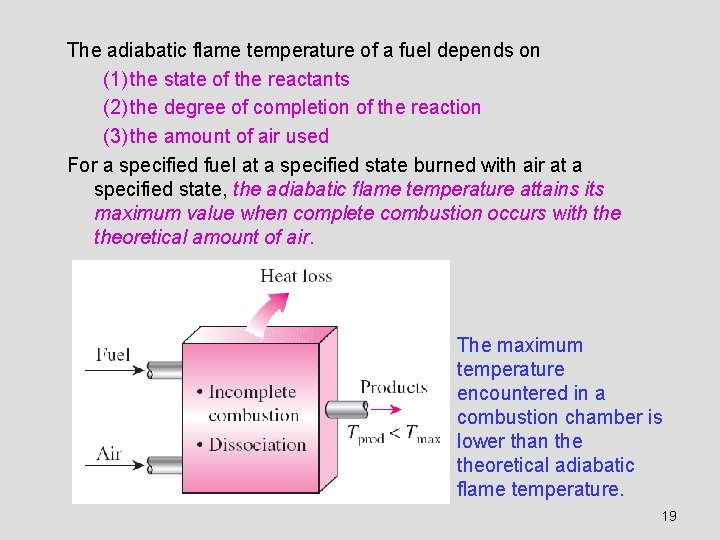The adiabatic flame temperature of a fuel depends on (1) the state of the reactants (2) the degree of completion of the reaction (3) the amount of air used For a specified fuel at a specified state burned with air at a specified state, the adiabatic flame temperature attains its maximum value when complete combustion occurs with theoretical amount of air. The maximum temperature encountered in a combustion chamber is lower than theoretical adiabatic flame temperature. 19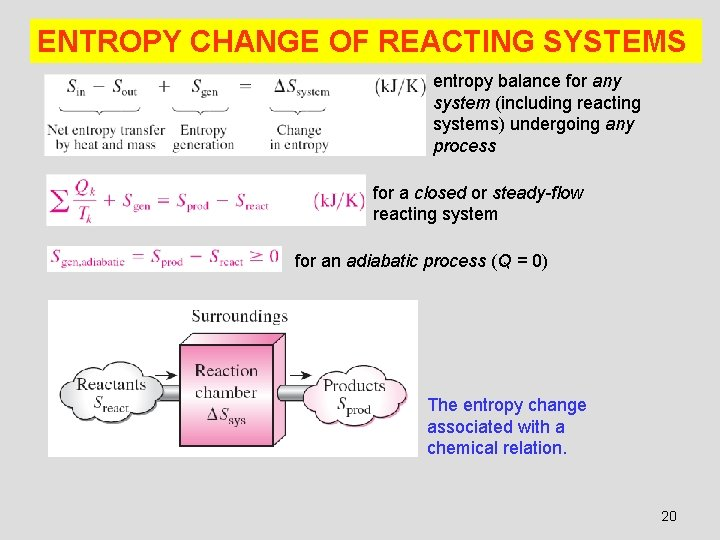ENTROPY CHANGE OF REACTING SYSTEMS entropy balance for any system (including reacting systems) undergoing any process for a closed or steady-flow reacting system for an adiabatic process (Q = 0) The entropy change associated with a chemical relation. 20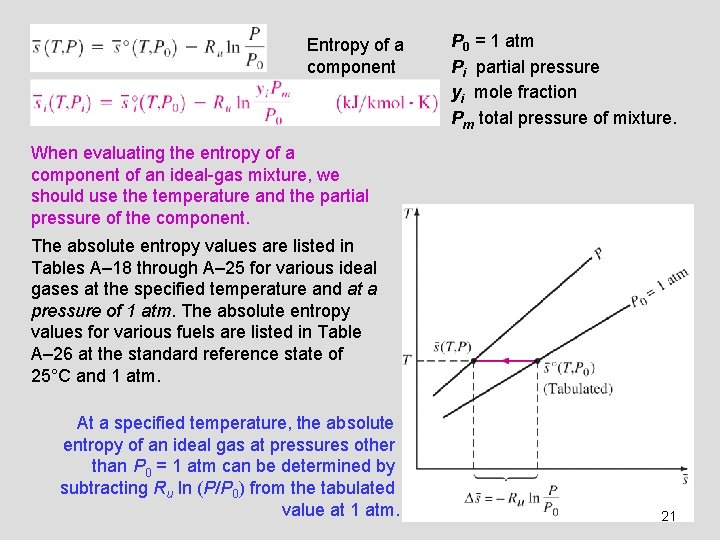Entropy of a component P 0 = 1 atm Pi partial pressure yi mole fraction Pm total pressure of mixture. When evaluating the entropy of a component of an ideal-gas mixture, we should use the temperature and the partial pressure of the component. The absolute entropy values are listed in Tables A– 18 through A– 25 for various ideal gases at the specified temperature and at a pressure of 1 atm. The absolute entropy values for various fuels are listed in Table A– 26 at the standard reference state of 25°C and 1 atm. At a specified temperature, the absolute entropy of an ideal gas at pressures other than P 0 = 1 atm can be determined by subtracting Ru ln (P/P 0) from the tabulated value at 1 atm. 21SECOND-LAW ANALYSIS OF REACTING SYSTEMS Exergy destruction The reversible work for a steady-flow combustion process that involves heat transfer with only the surroundings at T 0 When both the reactants and the products are at T 0 Gibbs function The difference between the exergy of the reactants and of the products during a chemical reaction is the reversible work associated with that reaction. 22For the very special case of Treact = Tprod = T 0 = 25°C We can conclude from the above equation that the value (the negative of the Gibbs function of formation at 25°C and 1 atm) of a compound represents the reversible work associated with the formation of that compound from its stable elements at 25°C and 1 atm in an environment at 25°C and 1 atm. 23Summary • Fuels and combustion • Theoretical and actual combustion processes • Enthalpy of formation and enthalpy of combustion • First-law analysis of reacting systems ü Steady-flow systems ü Closed systems • Adiabatic flame temperature • Entropy change of reacting systems • Second-law analysis of reacting systems 24